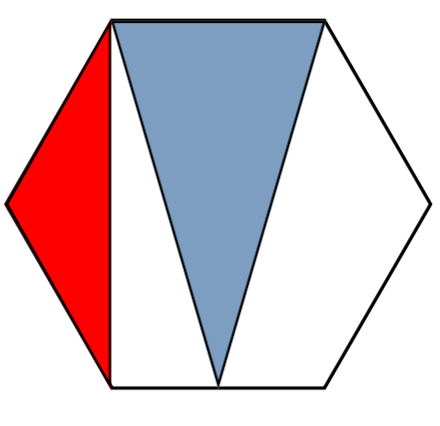# Red vs. Blue: The Hexagon Edition

Geometry Level 3

Given the regular hexagon below, the ratio of the red area to the blue area can be expressed as $\frac{A}{B}$ where $A$ and $B$ are coprime.Find $B-A$.

×

Problem Loading...

Note Loading...

Set Loading...